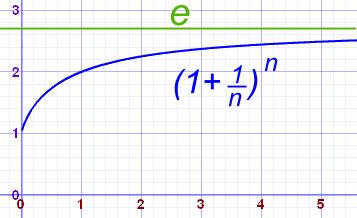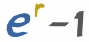* indicates a required field• phone number format invalid• email format invalidProblems detected, please review the form.
Privacy - Terms

# News from Mathnasium of Pflugerville

### e (Euler's Number)

Aug 14, 2017The number e is a famous irrational number, and is one of the most important numbers in mathematics.

The first few digits are:

2.7182818284590452353602874713527 (and more ...)

It is often called Euler's number after Leonhard Euler.

And Euler is spoken like "Oiler".

e is the base of the Natural Logarithms (invented by John Napier).

e is found in many interesting areas, so it is worth learning about.

## Calculating

There are many ways of calculating the value of e, but none of them ever give an exact answer, because e is irrational (not the ratio of two integers).

But it is known to over 1 trillion digits of accuracy!

For example, the value of (1 + 1/n)n approaches e as n gets bigger and bigger:n (1 + 1/n)n 1 2.00000 2 2.25000 5 2.48832 10 2.59374 100 2.70481 1,000 2.71692 10,000 2.71815 100,000 2.71827

## Another Calculation

The value of e is also equal to 1/0! + 1/1! + 1/2! + 1/3! + 1/4! + 1/5! + 1/6! + 1/7! + ... (etc)

(Note: "!" means factorial)

The first few terms add up to: 1 + 1 + 1/2 + 1/6 + 1/24 + 1/120 = 2.718055556

And you can try that yourself at Sigma Calculator.

## Remembering

To remember the value of e (to 10 places) just remember this saying (count the letters!):

• To
• express
• e
• remember
• to
• memorize
• a
• sentence
• to
• simplify
• this

Or you can remember the curious pattern that after the "2.7" the number "1828" appears TWICE:

2.7 1828 1828

And following THAT are the angles 45°, 90°, 45° in a Right-Angled Isosceles (two equal angles) Triangle:

2.7 1828 1828 45 90 45

(An instant way to seem really smart!)

## An Interesting Property

### Just for fun, try "Cut Up Then Multiply"

Let us say that we cut a number into equal parts and then multiply those parts together.

### Example: Cut 20 into 4 pieces and multiply them:

Each "piece" is 20/4 = 5 in size

5×5×5×5 = 54 = 625

Now, ... how could we get the answer to be as big as possible, what size should each piece be?

### Example continued: try 5 pieces

Each "piece" is 20/5 = 4 in size

4×4×4×4×4 = 45 = 1024

Yes, the answer is bigger! But is there a best size?

The answer: make the parts "e" (or as close to e as possible) in size.

### Example: 10

 10 cut into 3 parts is 3.3... 3.3...×3.3...×3.3... (3.3...)3 = 37.037... 10 cut into 4 equal parts is 2.5 2.5×2.5×2.5×2.5 = 2.54 = 39.0625 10 cut into 5 equal parts is 2 2×2×2×2×2 = 25 = 32

The winner is the number closest to "e", in this case 2.5.

Try it with another number yourself, say 100, ... what do you get?

## Advanced: Use of e in Compound Interest

Often the number e appears in unexpected places.

For example, e is used in Continuous Compounding (for loans and investments):Formula for Continuous Compounding

### Why does that happen?

Well, the formula for Periodic Compounding is:

FV = PV (1+r/n)n

where FV = Future Value
PV = Present Value
r = annual interest rate (as a decimal)
n = number of periods

But what happens when the number of periods heads to infinity?

The answer lies in the similarity between:

 (1+r/n)n and (1 + 1/n)n Compounding Formula e (as n approaches infinity)

By substituting x = n/r :

• r/n becomes 1/x and
• n becomes xr

And so:

 (1+r/n)n becomes (1+(1/x))xr

Which is just like the formula for e (as n approaches infinity), with an extra r as an exponent.

So, as x goes to infinity, then (1+(1/x))xr goes to er

And that is why e makes an appearance in interest calculations!